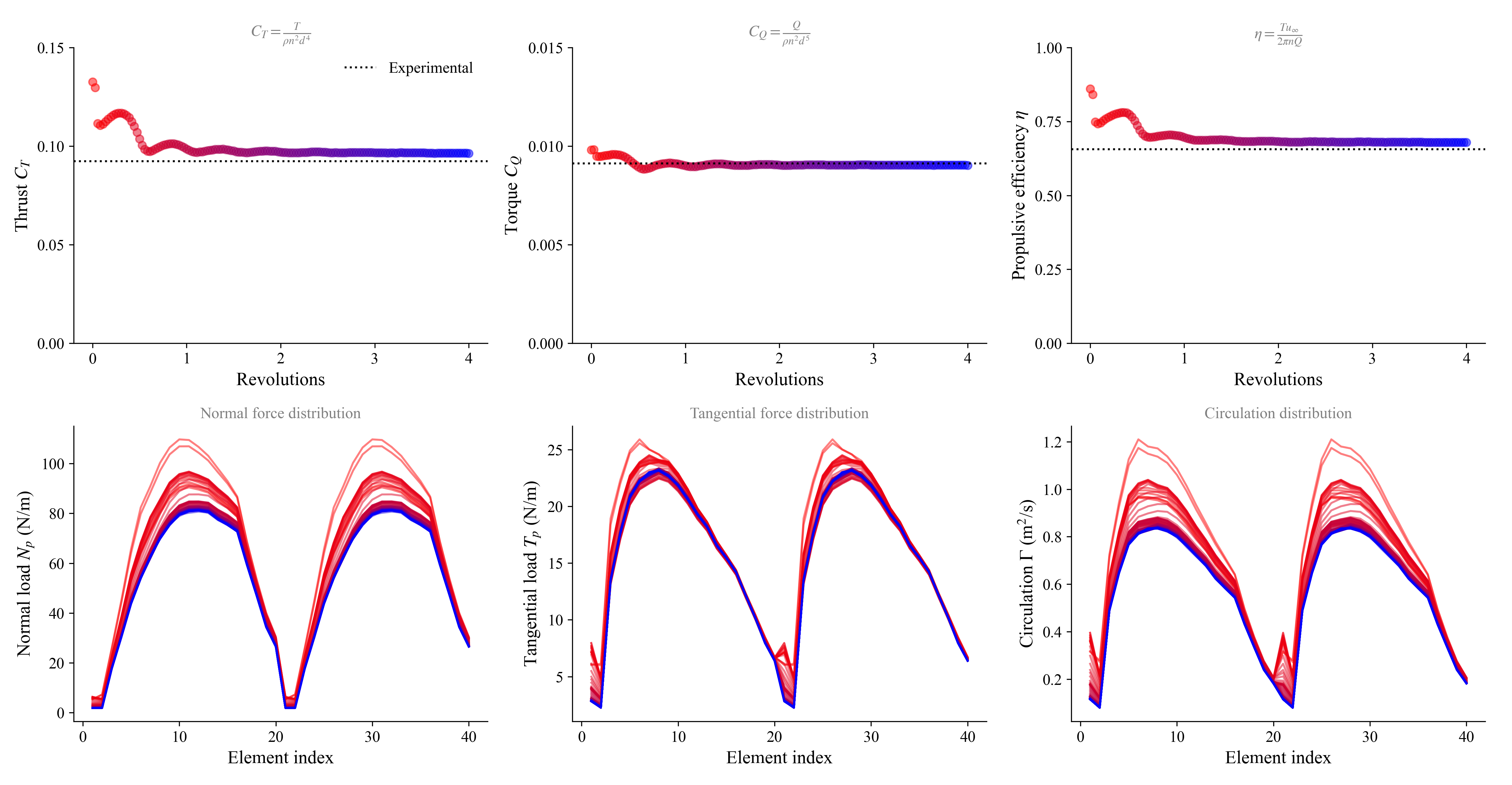# BasicsIn this example we first simulate an APC Thin-Electric 10x7 propeller operating in cruise conditions. Along the way, we demonstrate the basics of how to set up and run a rotor simulation.

Rotors are generated through the function FLOWUnsteady.generate_rotor, which can receive either a set of parameters that define the rotor geometry (like twist/chord/sweep distributions, etc), or it can read the rotor geometry from a file. FLOWunsteady provides a prepopulated database of airfoil and rotor geometries to automate the generation of rotors, which is found under database/. This database can be accessed through the variable FLOWUnsteady.default_database. Alternatively, users can define their own database with custom rotors and airfoils.

The following slides describe the structure of the database, using a DJI rotor as an example:

In this simulation we exemplify the following:

#=##############################################################################
# DESCRIPTION
Simulation of an APC Thin-Electric 10x7 propeller (two-bladed rotor, 10-inch
diameter).

This example replicates the experiment and simulation described in McCrink &
Gregory (2017), "Blade Element Momentum Modeling of Low-Reynolds Electric
Propulsion Systems."

# AUTHORSHIP
* Author          : Eduardo J. Alvarez (edoalvarez.com)
* Email           : Edo.AlvarezR@gmail.com
* Created         : Mar 2023
* Last updated    : Mar 2023
=###############################################################################

import FLOWVLM as vlm
import FLOWVPM as vpm

run_name        = "propeller-example"       # Name of this simulation

save_path       = run_name                  # Where to save this simulation
paraview        = true                      # Whether to visualize with Paraview

# ----------------- GEOMETRY PARAMETERS ----------------------------------------

# Rotor geometry
rotor_file      = "apc10x7.csv"             # Rotor geometry
data_path       = uns.def_data_path         # Path to rotor database
pitch           = 0.0                       # (deg) collective pitch of blades
CW              = false                     # Clock-wise rotation
xfoil           = true                      # Whether to run XFOIL
ncrit           = 9                         # Turbulence criterion for XFOIL

# NOTE: If xfoil=true, XFOIL will be run to generate the airfoil polars used
#       by blade elements before starting the simulation. XFOIL is run
#       on the airfoil contours found in rotor_file at the corresponding
#       local Reynolds and Mach numbers along the blade.
#       Alternatively, the user can provide pre-computer airfoil polars using
#       xfoil=false and pointing to polar files through rotor_file.

# Discretization
r               = 1/5                       # Geometric expansion of elements

# NOTE: Here a geometric expansion of 1/5 means that the spacing between the
#       tip elements is 1/5 of the spacing between the hub elements. Refine the
#       discretization towards the blade tip like this in order to better
#       resolve the tip vortex.

# ----------------- SIMULATION PARAMETERS --------------------------------------

# Operating conditions
RPM             = 9200                      # RPM
J               = 0.4                       # Advance ratio Vinf/(nD)
AOA             = 0                         # (deg) Angle of attack (incidence angle)

rho             = 1.225                     # (kg/m^3) air density
mu              = 1.81e-5                   # (kg/ms) air dynamic viscosity
speedofsound    = 342.35                    # (m/s) speed of sound

magVinf         = J*RPM/60*(2*R)
Vinf(X, t)      = magVinf*[cosd(AOA), sind(AOA), 0] # (m/s) freestream velocity vector

ReD             = 2*pi*RPM/60*R * rho/mu * 2*R      # Diameter-based Reynolds number
Matip           = 2*pi*RPM/60*R / speedofsound      # Tip Mach number

println("""
RPM:    $(RPM) Vinf:$(Vinf(zeros(3), 0)) m/s
Matip:  $(round(Matip, digits=3)) ReD:$(round(ReD, digits=0))
""")

# ----------------- SOLVER PARAMETERS ------------------------------------------

# Aerodynamic solver
VehicleType     = uns.UVLMVehicle           # Unsteady solver
# VehicleType   = uns.QVLMVehicle           # Quasi-steady solver
const_solution  = VehicleType==uns.QVLMVehicle  # Whether to assume that the
# solution is constant or not
# Time parameters
nrevs           = 4                         # Number of revolutions in simulation
nsteps_per_rev  = 36                        # Time steps per revolution
nsteps          = const_solution ? 2 : nrevs*nsteps_per_rev # Number of time steps
ttot            = nsteps/nsteps_per_rev / (RPM/60)       # (s) total simulation time

# VPM particle shedding
p_per_step      = 2                         # Sheds per time step
shed_starting   = true                      # Whether to shed starting vortex
max_particles   = ((2*n+1)*B)*nsteps*p_per_step + 1 # Maximum number of particles

# Regularization
sigma_rotor_surf= R/40                      # Rotor-on-VPM smoothing radius
lambda_vpm      = 2.125                     # VPM core overlap
sigma_vpm_overwrite = lambda_vpm * 2*pi*R/(nsteps_per_rev*p_per_step)

# Rotor solver
vlm_rlx         = 0.7                       # VLM relaxation <-- this also applied to rotors
hubtiploss_correction = vlm.hubtiploss_nocorrection # Hub and tip loss correction

# VPM solver
vpm_viscous     = vpm.Inviscid()            # VPM viscous diffusion scheme

# NOTE: In most practical situations, open rotors operate at a Reynolds number
#       high enough that viscous diffusion in the wake is negligible.
#       Hence, it does not make much of a difference whether we run the
#       simulation with viscous diffusion enabled or not.

if VehicleType == uns.QVLMVehicle
# NOTE: If the quasi-steady solver is used, this mutes warnings regarding
#       potential colinear vortex filaments. This is needed since the
#       quasi-steady solver will probe induced velocities at the lifting
uns.vlm.VLMSolver._mute_warning(true)
end

# ----------------- 1) VEHICLE DEFINITION --------------------------------------
println("Generating geometry...")

# Generate rotor
rotor = uns.generate_rotor(rotor_file; pitch=pitch,
altReD=[RPM, J, mu/rho],
xfoil=xfoil,
ncrit=ncrit,
data_path=data_path,
verbose=true,
verbose_xfoil=false,
plot_disc=true
);

println("Generating vehicle...")

# Generate vehicle
system = vlm.WingSystem()                   # System of all FLOWVLM objects

rotors = [rotor];                           # Defining this rotor as its own system
rotor_systems = (rotors, );                 # All systems of rotors

wake_system = vlm.WingSystem()              # System that will shed a VPM wake
# NOTE: Do NOT include rotor when using the quasi-steady solver
if VehicleType != uns.QVLMVehicle
end

vehicle = VehicleType(   system;
rotor_systems=rotor_systems,
wake_system=wake_system
);

# NOTE: Through the rotor_systems keyword argument to uns.VLMVehicle we
#       have declared any systems (groups) of rotors that share a common RPM.
#       We will later declare the control inputs to each rotor system when we
#       define the uns.KinematicManeuver.

# ------------- 2) MANEUVER DEFINITION -----------------------------------------
# Non-dimensional translational velocity of vehicle over time
Vvehicle(t) = zeros(3)

# Angle of the vehicle over time
anglevehicle(t) = zeros(3)

# RPM control input over time (RPM over RPMref)
RPMcontrol(t) = 1.0

angles = ()                                 # Angle of each tilting system (none)
RPMs = (RPMcontrol, )                       # RPM of each rotor system

maneuver = uns.KinematicManeuver(angles, RPMs, Vvehicle, anglevehicle)

# NOTE: FLOWUnsteady.KinematicManeuver defines a maneuver with prescribed
#       kinematics. Vvehicle defines the velocity of the vehicle (a vector)
#       over time. anglevehicle defines the attitude of the vehicle over time.
#       angle defines the tilting angle of each tilting system over time.
#       RPM defines the RPM of each rotor system over time.
#       Each of these functions receives a nondimensional time t, which is the
#       simulation time normalized by the total time ttot, from 0 to
#       1, beginning to end of simulation. They all return a nondimensional
#       output that is then scaled by either a reference velocity (Vref) or
#       a reference RPM (RPMref). Defining the kinematics and controls of the
#       maneuver in this way allows the user to have more control over how fast
#       to perform the maneuver, since the total time, reference velocity and
#       RPM are then defined in the simulation parameters shown below.

# ------------- 3) SIMULATION DEFINITION ---------------------------------------

Vref = 0.0                                  # Reference velocity to scale maneuver by
RPMref = RPM                                # Reference RPM to scale maneuver by

Vinit = Vref*Vvehicle(0)                    # Initial vehicle velocity
Winit = pi/180*(anglevehicle(1e-6) - anglevehicle(0))/(1e-6*ttot)  # Initial angular velocity

simulation = uns.Simulation(vehicle, maneuver, Vref, RPMref, ttot;
Vinit=Vinit, Winit=Winit);

# ------------- 4) MONITORS DEFINITIONS ----------------------------------------
figs, figaxs = [], []                       # Figures generated by monitor

# Generate rotor monitor
monitor_rotor = uns.generate_monitor_rotors(rotors, J, rho, RPM, nsteps;
t_scale=RPM/60,        # Scaling factor for time in plots
t_lbl="Revolutions",   # Label for time axis
out_figs=figs,
out_figaxs=figaxs,
save_path=save_path,
run_name=run_name,
figname="rotor monitor",
)

# ------------- 5) RUN SIMULATION ----------------------------------------------
println("Running simulation...")

uns.run_simulation(simulation, nsteps;
# ----- SIMULATION OPTIONS -------------
Vinf=Vinf,
rho=rho, mu=mu, sound_spd=speedofsound,
# ----- SOLVERS OPTIONS ----------------
p_per_step=p_per_step,
max_particles=max_particles,
vpm_viscous=vpm_viscous,
sigma_vlm_surf=sigma_rotor_surf,
sigma_rotor_surf=sigma_rotor_surf,
sigma_vpm_overwrite=sigma_vpm_overwrite,
vlm_rlx=vlm_rlx,
hubtiploss_correction=hubtiploss_correction,
shed_starting=shed_starting,
extra_runtime_function=monitor_rotor,
# ----- OUTPUT OPTIONS ------------------
save_path=save_path,
run_name=run_name,
);

# ----------------- 6) VISUALIZATION -------------------------------------------
if paraview
println("Calling Paraview...")

# Files to open in Paraview
files = joinpath(save_path, run_name*"_pfield...xmf;")
for bi in 1:B
global files
files *= run_name*"_Rotor_Blade$(bi)_loft...vtk;" files *= run_name*"_Rotor_Blade$(bi)_vlm...vtk;"
end

# Call Paraview
run(paraview --data=\$(files))

end


Run time: ~2 minutes on a Dell Precision 7760 laptop.

As the simulation runs, you will see the monitor shown below plotting the blade loading along with thrust and torque coefficients and propulsive efficiency.(red = beginning, blue = end)